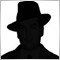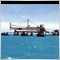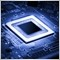• Overview
• Reviews (6)

# ImpMA

The standard indicator Moving Average is calculated on the basis of 7 price constants only. This modification allows defining Moving Average on the basis of any combination of 4 basic prices: Close, Open, High, and Low.

The additional convenience of the indicator is the possibility to choose from 6 methods of averaging: simple (SMA), exponential (EMA), smoothed (SMMA), linear weighted (LWMA), double exponential (DEMA), triple exponential (TEMA), and displaying on the basis of another timeframe.

Input parameters:
• TimeFrame - timeframe to display the indicator;
• Period - averaging period for calculation;
• Shift - shift of the indicator relative to the chart;
• Moving average mode - averaging method: Simple (SMA), Exponential (EMA), Smoothed (SMMA), Linear Weighted (LWMA), Double Exponential (DEMA), Triple Exponential (TEMA).
• Close - weight coefficient of the CLOSE price for calculation of the individual price;
• Open - weight coefficient of the OPEN price for calculation of the individual price;
• High - weight coefficient of the HIGH price for calculation of the individual price;
• Low - weight coefficient of the LOW price for calculation of the individual price.

Example 1. Calculate indicator by OPEN price: Close=0, Open=1, High=0, Low=0.

Example 2. Calculate indicator by WEIGHTED price: Close=2, Open=0, High=1, Low=1.

Example 3. Calculate indicator by individual price constant: Close=6, Open=7, High=1, Low=3. Price=(6*Close + 7*Open + 1*High + 3*Low)/17.16477
Nikolay Gaylis 2019.01.14 08:15

User didn't leave any comment to the rating12010
2018.02.02 20:15

User didn't leave any comment to the rating5631
2017.11.09 14:54

User didn't leave any comment to the rating6158
2017.07.21 18:34

User didn't leave any comment to the rating7173
2016.03.12 21:56

User didn't leave any comment to the rating7826
2014.04.08 12:35

User didn't leave any comment to the rating